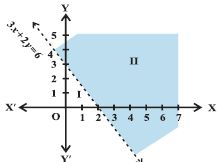Email us to get an instant 20% discount on highly effective K-12 Math & English kwizNET Programs!

#### Online Quiz (WorksheetABCD)

Questions Per Quiz = 2 4 6 8 10

### High School Mathematics - 2 Graphical Representation of Solutions to Inequalities

 Example: Solve 3x+2y> 6 graphically. Solution: The dotted line is the graph of the given inequality.This dotted line divides the xy plane in two half planes I and II. We select a point say (0,0) which lies in one of the half planes. We determine if this point satisfies the give inequality 3(0) + 2(0) > 6 which is false. The shaded half plane II excluding the points on the line is the solution region of the inequality. Directions: Draw the graphs.